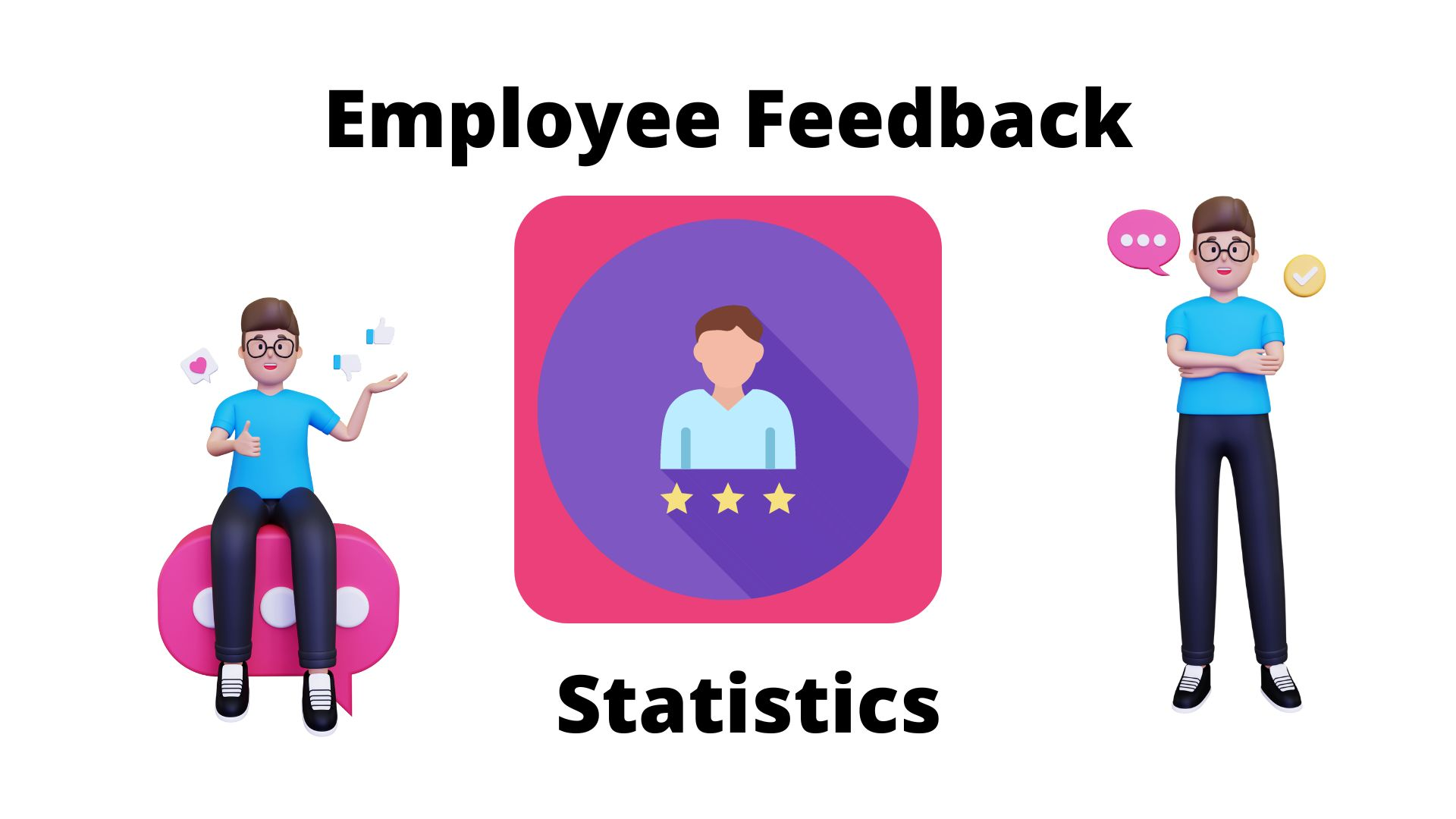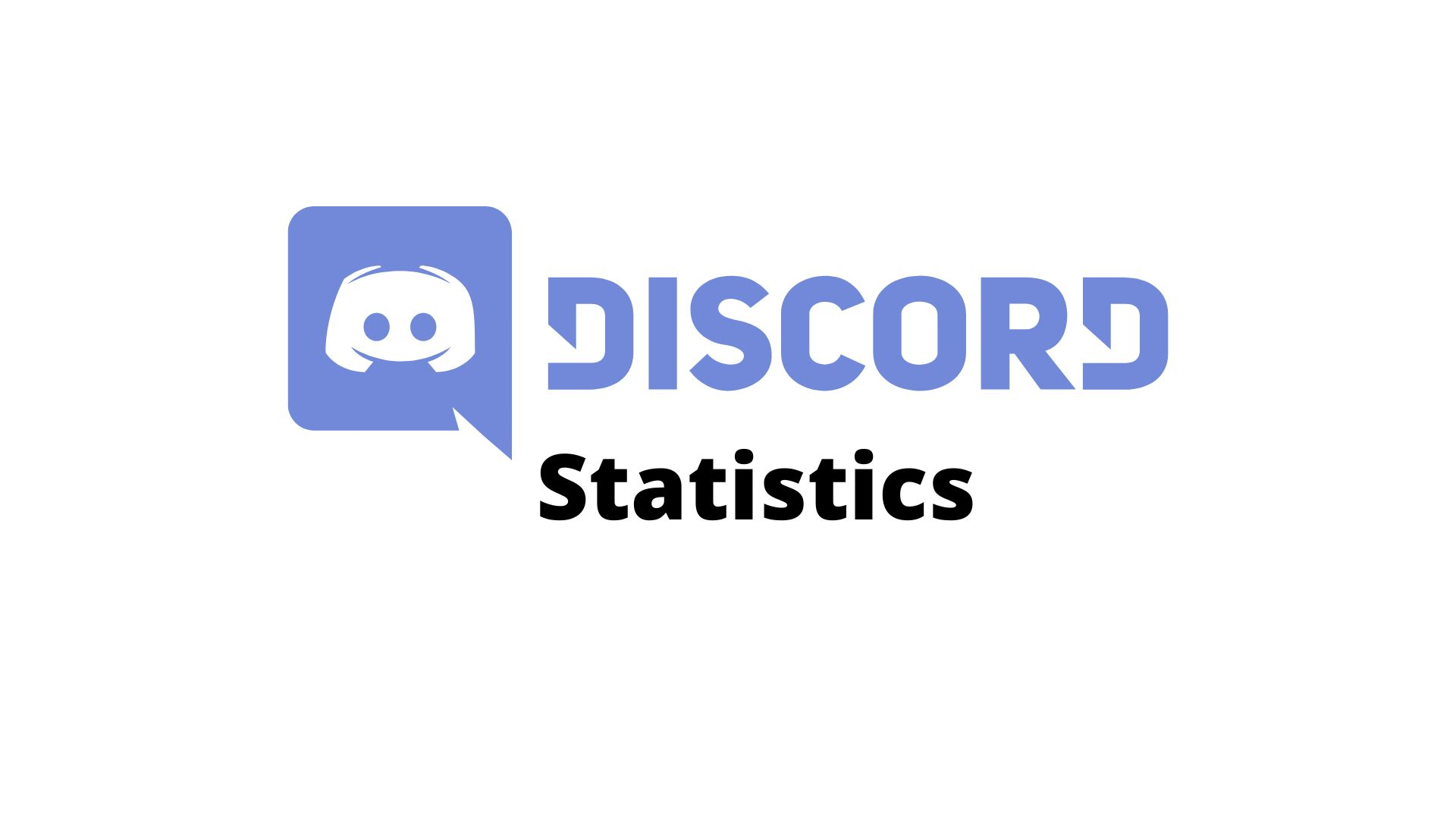# Descriptive VS Inferential StatisticsBarry Elad

Updated · Sep 08, 2023## Introduction

Understanding Descriptive VS Inferential Statistics, descriptive statistics are summary statistics that can help to understand the individual quantitative observations and the general process to retrieve the insights from the data. This is only a descriptive method and thus does not focus on the differences between two variables

## Descriptive VS Inferential Statistics

### Descriptive Statistics

• Descriptive statistics can be easily presented using graphics, tables, or charts.
• This method is used to describe a situation.
• As the name suggests, it explains already-known information.
• Moreover, descriptive statistics provide shape to the given raw data.
• In this case, organization and analysis of the raw data are easy.
• The given data is limited to a sample only.

### Inferential Statistics

• Inferential Statistics are used to understand the occurrence of a case in an event.
• As the same says, inferences can be drawn using the data of the population.
• The results in this case are retrieved on the basis of probability.
• Moreover, the results almost provide the conclusion on the population.
• Comparison between different datasets based on predictions can be successfully performed.

## Types of Descriptive Statistics

Variability: Also called a dispersion in a dataset, the way the values are spread around.  The central tendency in the set of data helps to understand the variability. Moreover, it includes a wide range of measurements. Variability has some commonly used measures as follows:

• Skewness: Skewness is a measurement of symmetry in a given dataset. If the left-hand curve is longer than the right one and seen as a flat curve then the value is negative. On the other hand, when the right curve is long and flat then it is seen as positive.
• Range: In order to understand the size of the distribution of values, the smallest value is often subtracted from the largest value.
• Standard deviation: The quantity of variation or dispersion can be known using standard deviation. For the values which are nearby the mean value, a low standard deviation is implied. On the other hand, values spread across are referred to as high standard deviation.
• Kurtosis: To understand whether the data include extreme values Kurtosis analysis is performed. The extreme values are also referred to as Outliners. If the provided data includes a high range of extreme values, then we call it a high Kurtosis.
• Minimum & maximum values: These values represent the highest and lowest values in the datasets.
• Central Tendency: The common central values in a given dataset for measurements are referred to as a central tendency.  But, instead of giving a reference to the whole dataset, it only focuses on a variety of central measurements. Some measures of central tendency include:
• Mode: refers to the commonly observed value in the dataset.
• Median: The middle value in the given dataset.
• Mean: The average value in the entire dataset.
• Distribution: Distribution explains the frequency of different results in the given dataset. Values of the results in the distribution category can be presented using a graph, table, and list. Visual representation is a common practice in descriptive statistics.

## Inferential Statistics

In Addition, Descriptive VS Inferential Statistics are based on two different bases as one method provides more generalized results while the other does the opposite. Inferential Statistics, therefore, depends on general results based on a larger population. Moreover, this method is based on probability rather than facts. The accuracy of the results obtained is based on the accuracy and representative of the larger population. Therefore, the results are gained from the random sample. Observations in the random samples with biased meaning are not considered.  Random sampling is a crucial step to perform inferential Statistics.

Random Sampling involves the factors such as defining the population, deciding the sample size, drawing a sample, and analyzing. Additionally, other techniques include Confidence intervals, hypothesis testing, and regression and correlation analysis.

## Conclusion

Concluding the Descriptive VS Inferential Statistics, even though these are two different statistics and the results given are the opposites of each other, using Descriptive and Inferential Statistics collectively provides accurate results as these are together called powerful statistical techniques. On the other hand, using only descriptive statistics, won’t come in handy for decision-making.

### FAQ.

How to Calculate Descriptive Statistics in excel?

Descriptive Statistics helps to analyze data such as mean, median, mode, standard deviation, and quartiles. With excessive data on one hand, Excel makes it easy to calculate the above functions.

Let’s assume the numbers in excel as:

A1:3

A2:18

A3:90

A4:17

A5:35

A6:8

For calculating the average function use =AVERAGE(range). If we want to take an average between 3 to 17 then the calculation would be =AVERAGE(A1:A4).

For calculating the mode function use =MODE(A1:A4).

For calculating the standard deviation function use =STDEV(A1:A4).

For calculating the median function use =MEDIAN(A1:A4).

For calculating the QUARTILE function use =QUARTILE(A1:A4).

If you want to calculate more columns use, between two datasets. For eg. (A1:A4, B1:B4)

For calculating the mode function use

Therefore =AVERGAE(

What is the purpose of Descriptive statistics?

The purpose of descriptive statistics is to give information about the data set.

What are the types of descriptive statistics?

There are three types of descriptive statistics, involving, frequency distribution, central tendency, and variability of the data. In simple words, frequency distribution measures the common occurrence of the data, central tendency measures the midpoint of distribution, and degree of dispersion is measured by the variability of data.

Explain Descriptive VS Inferential Statistics in simple words.

Descriptive Statistics deals with the collection, analysis, interpretation, and presentation of the given data in a descriptive way. On the other hand, inferential statistics predicts inferences on a given dataset of the larger population.

What is the population in Statistics?

Population in terms of statistics includes an observation of individuals irrespective of the nation, group of people along with their common features, and also the group of individuals.Barry is a lover of everything technology. Figuring out how the software works and creating content to shed more light on the value it offers users is his favorite pastime. When not evaluating apps or programs, he's busy trying out new healthy recipes, doing yoga, meditating, or taking nature walks with his little one.